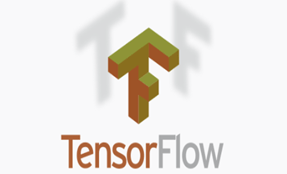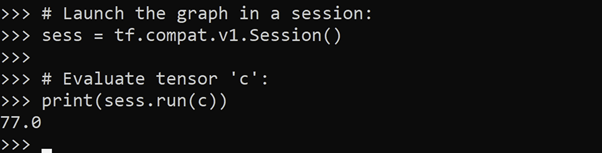# What is TensorFlowThis Python tutorial is a part of our series of Python packages related tutorials.
Try a faster and easier way to manage your Python packages and resolve dependencies. Use Python 3.9 by ActiveState and build your own runtime with the packages and dependencies you need. The ActiveState Platform’s command line interface, the State Tool will automatically resolve dependencies for you to ensure your Python environment won’t be corrupted by installing incompatible dependencies.

Get started for free by creating an account on the ActiveState Platform or logging in with your GitHub account.

## What is TensorFlow

TensorFlow is an open source Python library for complex numeric computation. It is a foundation library that can be used to create Machine Learning/Deep Learning neural network models, such as:

• Differentiable neural networks
• Deep network models that require gradient optimization

###TensorFlow is an open source Python library for complex numeric computation. It is a foundation library that can be used to create Machine Learning/Deep Learning neural network models, such as:

• Differentiable neural networks
• Deep network models that require gradient optimization

TensorFlow’s low-level APIs can serve as the backend computational engine for a high level interface like Keras, which simplifies the definition and training of network models. For more information about Keras, refer to What is a Keras Model.

TensorFlow combines:

• Symbolic Math. Use of computers to calculate mathematical equations and expressions in symbolic form, eg. x*y
• Dataflow. Disconnection of data components into data pipelines that execute concurrently.
• Differentiable Programming. Gradient based optimization involving differential functions.
• Generalization. In TensorFlow, a tensor is an abstraction over a multidimensional array. The essential meaning of a tensor as defined in tensor calculus is kept, but the formalism and details of tensor calculus are dropped.

TensorFlow sacrifices user-friendless for power:

• Efficient computation of mathematical expressions containing multi-dimensional arrays.
• Handles difficult, complex algorithms and models that other platforms cannot handle.
• Flexible enough for use cases requiring custom coding, yet able to automate many tasks with built-in algorithms and pre-trained models.
• Highly scalable from single machines to distributed networks with huge datasets.
• Can utilize CPU, TPU (Tensor Processing Unit), and GPU (Graphics Processing Unit) to perform computations.

### Start Using TensorFlow

If you don’t have TensorFlow installed, refer to How To Install Keras And TensorFlow.

The easiest way to start working with TensorFlow is by using Keras, which integrates seamlessly as a high level interface for TensorFlow. The best way is to begin with the Keras Sequential API, and then try its Functional API. Once you are familiar with Keras (and its limitations), you can consider the more comprehensive TensorFlow platform.

### TensorFlow Keras Example

In this example, a TensorFlow Keras application is created by combining the TensorFlow – foundation library with Keras classes (TensorFlow subclasses). We’ll define a simple CNN (Convolutional Neural Network) model for handwritten character recognition using the MNIST dataset:

```# Import the required TensorFlow library
# and Keras classes for this example:
import tensorflow as tf
from tensorflow.keras import Model
from tensorflow.keras.models import Sequential
from tensorflow.keras.layers import Dense, Flatten, Conv2D, AveragePooling2D
# Load MNIST dataset and split into training and testing samples:
mnist = tf.keras.datasets.mnist
# Reshape the image dimensions:
x_train = x_train.reshape(x_train.shape, 28, 28, 1)
x_test = x_test.reshape(x_test.shape, 28, 28, 1)
# Normalize the output from the previous function:
x_train = tf.keras.utils.normalize(x_train, axis=1)
x_test = tf.keras.utils.normalize(x_test, axis=1)
# Build the model:
model = Sequential()
# Print a summary of the model:
model.summary()```

### TensorFlow Core Application Example

TensorFlow core applications typically consist of two discrete sections:

1. Construction Phase. Creation of a computational graph consisting of nodes (ops) and tensors (node ‘edges’).
2. Execution Phase. The graph is encapsulated in a session and run.

In this example, we construct a basic computational graph and execute it in a session:

```# Import the library required in this example:
import tensorflow as tf
# Class environment in which a graph will be created
# and run in a session:
tf.compat.v1.Session(
target='', graph=None, config=None
)
# Disable eager execution (immediate execution of operations
# as they are called) in TensorFlow 2.x:
tf.compat.v1.disable_eager_execution()
# Build a graph.
a = tf.constant(7.0)
b = tf.constant(11.0)
c = a * b
# Launch the graph in a session:
sess = tf.compat.v1.Session()
# Evaluate tensor 'c':
print(sess.run(c))```

Figure 1. Launch the graph in a session, and evaluate the tensor:### A modern solution to Python package management – Try ActiveState’s Platform

The ActiveState Platform is a cloud-based build tool for Python. It provides build automation and vulnerability remediation for:

• Python language cores, including Python 2.7 and Python 3.5+
• Python packages and their dependencies, including:
• Transitive dependencies (ie., dependencies of dependencies)
• Linked C and Fortran libraries, so you can build data science packages
• Operating system-level dependencies for Windows, Linux, and macOS
• Shared dependencies (ie., OpenSSL)
• Find, fix and automatically rebuild a secure version of Python packages like Django and environments in minutes#### Python Package Management In Action

Get a hands-on appreciation for how the ActiveState Platform can help you manage your dependencies for Python environments. Just run the following command to install Python 3.9 and our package manager, the State Tool:

Windows

`powershell -Command "& \$([scriptblock]::Create((New-Object Net.WebClient).DownloadString('https://platform.activestate.com/dl/cli/install.ps1'))) -activate-default ActiveState-Labs/Python-3.9Beta"`

Linux

`sh <(curl -q https://platform.activestate.com/dl/cli/install.sh) --activate-default ActiveState-Labs/Python-3.9Beta`